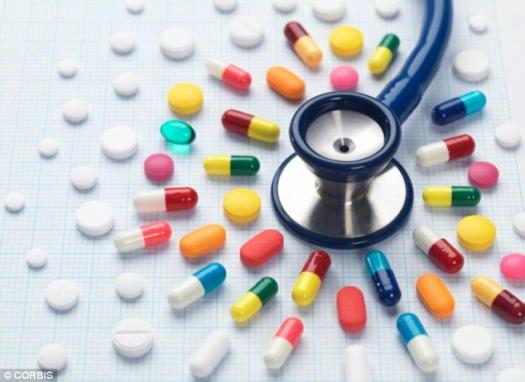# Medication Calculation

100 Questions | Total Attempts: 226SettingsIHNA EPIQ Medication Calculation

Related Topics
• 1.
The dose to be given is 4 g. The stock solution contains 160 mg per 5 ml. How many ml would you give?
• 2.
The dose to be given is 1.5 g. The stock solution contains 125 mg per 2 ml. How many ml would you give?
• 3.
The dose to be given is 75g. The stock solution contains 500 mg/2 ml. How many ml would you give?
• 4.
The dose to be given is 100mg. The stock solution contains 0.3g/6 ml. How many ml would you give?
• 5.
The total volume to be given is 4800 ml. The time over which this is to be given is 12 hours. How many ml per hour would you give?
• 6.
The total volume to be given is 2400 ml. The time over which this is to be given is 24 hours. How many ml per hour would you give?
• 7.
The total volume to be given is 120 ml. The time over which this is to be given is 2 hours. How many ml per hour would you give?
• 8.
The total volume to be given is 3600 ml. The time over which this is to be given is 24 hours. How many ml per hour would you give?
• 9.
The total volume to be given is 400 ml. The time over which this is to be given is 4 hours. How many ml per hour would you give?
• 10.
Your client weighs 60kg. The doctor has ordered a single dose of 2 mg/kg of Medication G. This medication is provided as 60mg in 2 ml. Calculate the volume to be administered.
• 11.
Mrs H weighs 70kg. She is to have 5mg/kg of Medication J. Medication J is supplied as 500mg/5ml. Calculate the volume to be administered.
• 12.
The PCA machine holds 50ml, containing 500mg of Medication P. The doctor has ordered a bolus dose of 50mg. Calculate the volume to be delivered.
• 13.
100 Grams of Medication A is added to 1 Litre of 0.9% saline. The dose prescribed is 5 Grams per hour. Calculate the rate of infusion in ml/hr.
• 14.
25,000 units of Heparin has been added to 500ml of Normal Saline. The dose prescribed is 600 units/hr. Calculate the rate of infusion in ml/hr.
• 15.
10,000 units of Heparin is added to 1 Litre of 0.9% Saline. Dose prescribed is 400 units per hour. Calculate the rate of infusion in ml/hr.
• 16.
Medication X is presented as 8,000 units in 1 ml. Dose required is 2,000 units. Calculate the volume to be administered.
• 17.
10,000 units of heparin have been added to 50 mls 0.9% saline for infusion via a syringe driver. The dose prescribed is 1,100 units per hour. Calculate the rate of infusion in mls per hour.
• 18.
30 mcg of Medication B has been ordered IV stat. The stock available is 0.03mg in 2ml. Calculate the volume to be administered.
• 19.
Medication C is supplied as 100mgs/2ml. The doctor has prescribed 50 mg, IM, 3-4/24. What is the volume to be administered?
• 20.
Medication D is supplied as 100 micrograms/ml. 0.15mg of Medication D, IM has been prescribed. Calculate the volume to be administered.
• 21.
240mg of Medication E elixir is to be administered. The stock from pharmacy comes as 120mg in 5 ml. Calculate the volume to be administered.
• 22.
Miss K weighs 60kg. She is to have 6mg/kg of Medication L. The stock available is 120mg/5ml. Calculate the volume to be administered.
• 23.
The order is for 187.5 mcg of Medication F. The stock available comes as 62.5mcg tablets. How many tablets will be administered?
• 24.
Amanda weighs 30kg. She is ordered 8mcg/kg of Medication C, 8/24. Calculate the amount of medication she will receive in 24 hours.
• 25.
You have been asked to care for a sixteen year old girl who has been admitted to the ward for an elective appendicectomy in the morning. At 2300 she asks for Nitrazepam and the order is for 10mg. Stock available is 0.005g. How many tablets should be given?